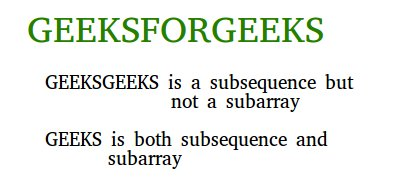Related Articles
Subarray/Substring vs Subsequence and Programs to Generate them
• Difficulty Level : Medium
• Last Updated : 09 Apr, 2021

Subarray/Substring

A subbarray is a contiguous part of array. An array that is inside another array. For example, consider the array [1, 2, 3, 4], There are 10 non-empty sub-arrays. The subbarays are (1), (2), (3), (4), (1,2), (2,3), (3,4), (1,2,3), (2,3,4) and (1,2,3,4). In general, for an array/string of size n, there are n*(n+1)/2 non-empty subarrays/subsrings.How to generate all subarrays?
We can run two nested loops, the outer loop picks starting element and inner loop considers all elements on right of the picked elements as ending element of subarray.

## C++

 `/*  C++ code to generate all possible subarrays/subArrays``    ``Complexity- O(n^3) */``#include``using` `namespace` `std;` `// Prints all subarrays in arr[0..n-1]``void` `subArray(``int` `arr[], ``int` `n)``{``    ``// Pick starting point``    ``for` `(``int` `i=0; i

## Java

 `// Java program toto generate all possible subarrays/subArrays``//  Complexity- O(n^3) */` `class` `Test``{``    ``static` `int` `arr[] = ``new` `int``[]{``1``, ``2``, ``3``, ``4``};``    ` `    ``// Prints all subarrays in arr[0..n-1]``    ``static` `void` `subArray( ``int` `n)``    ``{``        ``// Pick starting point``        ``for` `(``int` `i=``0``; i

## Python3

 `# Python3 code to generate all possible``# subarrays/subArrays``# Complexity- O(n^3)` `# Prints all subarrays in arr[0..n-1]``def` `subArray(arr, n):` `    ``# Pick starting point``    ``for` `i ``in` `range``(``0``,n):` `        ``# Pick ending point``        ``for` `j ``in` `range``(i,n):` `            ``# Print subarray between``            ``# current starting``            ``# and ending points``            ``for` `k ``in` `range``(i,j``+``1``):``                ``print` `(arr[k],end``=``" "``)` `            ``print` `(``"\n"``,end``=``"")` `            ` `# Driver program``arr ``=` `[``1``, ``2``, ``3``, ``4``]``n ``=` `len``(arr)``print` `(``"All Non-empty Subarrays"``)` `subArray(arr, n);` `# This code is contributed by Shreyanshi.`

## C#

 `// C# program toto generate all``// possible subarrays/subArrays``// Complexity- O(n^3)``using` `System;` `class` `GFG``{``    ``static` `int` `[]arr = ``new` `int``[]{1, 2, 3, 4};``    ` `    ``// Prints all subarrays in arr[0..n-1]``    ``static` `void` `subArray( ``int` `n)``    ``{``        ` `        ``// Pick starting point``        ``for` `(``int` `i = 0; i < n; i++)``        ``{``            ` `            ``// Pick ending point``            ``for` `(``int` `j = i; j < n; j++)``            ``{``                ` `                ``// Print subarray between current``                ``// starting and ending points``                ``for` `(``int` `k = i; k <= j; k++)``                    ``Console.Write(arr[k]+``" "``);``                    ``Console.WriteLine(``""``);``            ``}``        ``}``    ``}``    ` `    ``// Driver Code``    ``public` `static` `void` `Main()``    ``{``        ``Console.WriteLine(``"All Non-empty Subarrays"``);``        ``subArray(arr.Length);``        ` `    ``}` `}` `// This code is contributed by Sam007.`

## PHP

 ``

## Javascript

 ``

Output:

```All Non-empty Subarrays
1
1 2
1 2 3
1 2 3 4
2
2 3
2 3 4
3
3 4
4```

Subsequence
A subsequence is a sequence that can be derived from another sequence by zero or more elements, without changing the order of the remaining elements.
For the same example, there are 15 sub-sequences. They are (1), (2), (3), (4), (1,2), (1,3),(1,4), (2,3), (2,4), (3,4), (1,2,3), (1,2,4), (1,3,4), (2,3,4), (1,2,3,4). More generally, we can say that for a sequence of size n, we can have (2n-1) non-empty sub-sequences in total.
A string example to differentiate: Consider strings “geeksforgeeks” and “gks”. “gks” is a subsequence of “geeksforgeeks” but not a substring. “geeks” is both a subsequence and subarray. Every subarray is a subsequence. More specifically, Subsequence is a generalization of substring.

How to generate all Subsequences?
We can use algorithm to generate power set for generation of all subsequences.

## C++

 `/*  C++ code to generate all possible subsequences.``    ``Time Complexity O(n * 2^n) */``#include``using` `namespace` `std;` `void` `printSubsequences(``int` `arr[], ``int` `n)``{``    ``/* Number of subsequences is (2**n -1)*/``    ``unsigned ``int` `opsize = ``pow``(2, n);` `    ``/* Run from counter 000..1 to 111..1*/``    ``for` `(``int` `counter = 1; counter < opsize; counter++)``    ``{``        ``for` `(``int` `j = 0; j < n; j++)``        ``{``            ``/* Check if jth bit in the counter is set``                ``If set then print jth element from arr[] */``            ``if` `(counter & (1<

## Java

 `/*  Java code to generate all possible subsequences.``    ``Time Complexity O(n * 2^n) */` `import` `java.math.BigInteger;` `class` `Test``{``    ``static` `int` `arr[] = ``new` `int``[]{``1``, ``2``, ``3``, ``4``};``    ` `    ``static` `void` `printSubsequences(``int` `n)``    ``{``        ``/* Number of subsequences is (2**n -1)*/``        ``int` `opsize = (``int``)Math.pow(``2``, n);``     ` `        ``/* Run from counter 000..1 to 111..1*/``        ``for` `(``int` `counter = ``1``; counter < opsize; counter++)``        ``{``            ``for` `(``int` `j = ``0``; j < n; j++)``            ``{``                ``/* Check if jth bit in the counter is set``                    ``If set then print jth element from arr[] */``      ` `                ``if` `(BigInteger.valueOf(counter).testBit(j))``                    ``System.out.print(arr[j]+``" "``);``            ``}``            ``System.out.println();``        ``}``    ``}``    ` `    ``// Driver method to test the above function``    ``public` `static` `void` `main(String[] args)``    ``{``        ``System.out.println(``"All Non-empty Subsequences"``);``        ``printSubsequences(arr.length);``    ``}``}`

## Python3

 `# Python3 code to generate all``# possible subsequences.``# Time Complexity O(n * 2 ^ n)``import` `math` `def` `printSubsequences(arr, n) :` `    ``# Number of subsequences is (2**n -1)``    ``opsize ``=` `math.``pow``(``2``, n)` `    ``# Run from counter 000..1 to 111..1``    ``for` `counter ``in` `range``( ``1``, (``int``)(opsize)) :``        ``for` `j ``in` `range``(``0``, n) :``            ` `            ``# Check if jth bit in the counter``            ``# is set If set then print jth``            ``# element from arr[]``            ``if` `(counter & (``1``<

## C#

 `// C# code to generate all possible subsequences.``// Time Complexity O(n * 2^n)``using` `System;` `class` `GFG{` `static` `void` `printSubsequences(``int``[] arr, ``int` `n)``{``    ` `    ``// Number of subsequences is (2**n -1)``    ``int` `opsize = (``int``)Math.Pow(2, n);``    ` `    ``// Run from counter 000..1 to 111..1``    ``for``(``int` `counter = 1; counter < opsize; counter++)``    ``{``        ``for``(``int` `j = 0; j < n; j++)``        ``{``            ` `            ``// Check if jth bit in the counter is set``            ``// If set then print jth element from arr[]``            ``if` `((counter & (1 << j)) != 0)``                ``Console.Write(arr[j] + ``" "``);``        ``}``        ``Console.WriteLine();``    ``}``}` `// Driver Code``static` `void` `Main()``{``    ``int``[] arr = { 1, 2, 3, 4 };``    ``int` `n = arr.Length;``    ` `    ``Console.WriteLine(``"All Non-empty Subsequences"``);``    ` `    ``printSubsequences(arr, n);``}``}` `// This code is contributed by divyesh072019`

## PHP

 ``

## Javascript

 ``

Output:

```All Non-empty Subsequences
1
2
1 2
3
1 3
2 3
1 2 3
4
1 4
2 4
1 2 4
3 4
1 3 4
2 3 4
1 2 3 4```

This article is contributed by Harshit Gupta. If you like GeeksforGeeks and would like to contribute, you can also write an article and mail your article to contribute@geeksforgeeks.org. See your article appearing on the GeeksforGeeks main page and help other Geeks.
Please write comments if you find anything incorrect, or you want to share more information about the topic discussed above

Attention reader! Don’t stop learning now. Get hold of all the important DSA concepts with the DSA Self Paced Course at a student-friendly price and become industry ready.

My Personal Notes arrow_drop_up